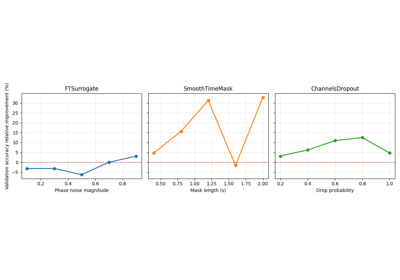# braindecode.augmentation.ChannelsDropout#

class braindecode.augmentation.ChannelsDropout(probability, p_drop=0.2, random_state=None)[source]#

Randomly set channels to flat signal.

Part of the CMSAugment policy proposed in 

Parameters
• probability (float) – Float setting the probability of applying the operation.

• proba_drop (float | None, optional) – Float between 0 and 1 setting the probability of dropping each channel. Defaults to 0.2.

• random_state (int | numpy.random.Generator, optional) – Seed to be used to instantiate numpy random number generator instance. Used to decide whether or not to transform given the probability argument and to sample channels to erase. Defaults to None.

References

1

Saeed, A., Grangier, D., Pietquin, O., & Zeghidour, N. (2020). Learning from Heterogeneous EEG Signals with Differentiable Channel Reordering. arXiv preprint arXiv:2010.13694.

Methods

get_augmentation_params(*batch)[source]#

Return transform parameters.

Parameters
• X (tensor.Tensor) – The data.

• y (tensor.Tensor) – The labels.

Returns

params – Contains

• p_dropfloat

Float between 0 and 1 setting the probability of dropping each channel.

• random_statenumpy.random.Generator

The generator to use.

Return type

dict

static operation(X, y, p_drop, random_state=None)#

Randomly set channels to flat signal.

Part of the CMSAugment policy proposed in 

Parameters
• X (torch.Tensor) – EEG input example or batch.

• y (torch.Tensor) – EEG labels for the example or batch.

• p_drop (float) – Float between 0 and 1 setting the probability of dropping each channel.

• random_state (int | numpy.random.Generator, optional) – Seed to be used to instantiate numpy random number generator instance. Defaults to None.

Returns

• torch.Tensor – Transformed inputs.

• torch.Tensor – Transformed labels.

References

1

Saeed, A., Grangier, D., Pietquin, O., & Zeghidour, N. (2020). Learning from Heterogeneous EEG Signals with Differentiable Channel Reordering. arXiv preprint arXiv:2010.13694.

## Examples using `braindecode.augmentation.ChannelsDropout`#Searching the best data augmentation on BCIC IV 2a Dataset

Searching the best data augmentation on BCIC IV 2a Dataset